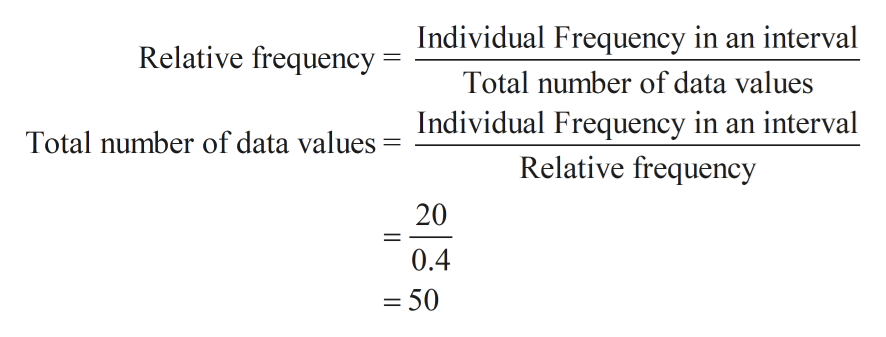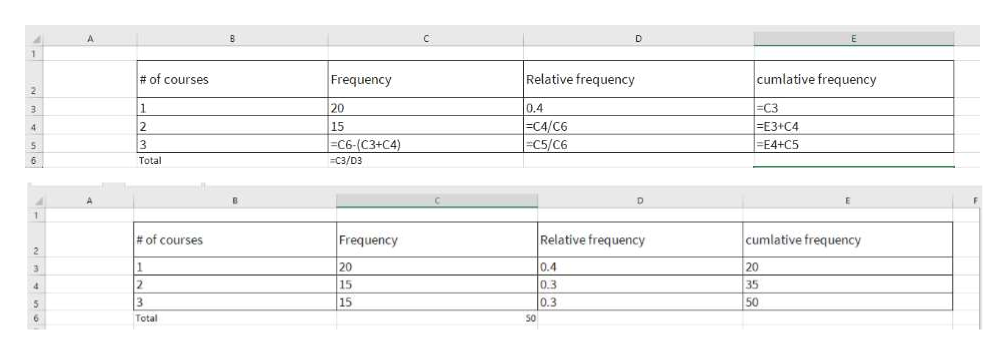# of coursesFrequencyRelative frequency cumlative frequancy 1200.4 215  3   What/ how do I fill in the blanks?

Question
 # of courses Frequency Relative frequency cumlative frequancy 1 20 0.4 2 15 3

What/ how do I fill in the blanks?

Step 1

Relative frequency can be calculated by dividing the individual frequency in an interval by total number of data values. As relative frequency for first interval is given along with individual frequency, total number of data values can be calculated as shown below.help_outlineImage TranscriptioncloseRelative frequency Individual Frequency in an interval Total number of data values Individual Frequency in an interval Relative frequency Total number of data values 20 0.4 - 50 || fullscreen
Step 2

Total number of data values have been calculated as 50.

We know that Sum of frequencies = Total number of data values

Therefore, last value under Frequency column can be calculated as 50 -(20+15) = 15

Remaining rel...help_outlineImage TranscriptioncloseD Relative frequency cumlative frequency # of courses Frequency 20 0.4 C4/C6 -C5/ C6 -C3 - E3+C4 E4+C5 1 3 2 15 C6-( C3+ C4 ) 3 5 C3/D3 6 Total 1 Relative frequency Frequency cumlative frequency # of courses 20 0.4 0.3 0.3 1 20 3 2 15 35 15 3 50 Total 50 fullscreen

Want to see the full answer?

See Solution

Want to see this answer and more?

Our solutions are written by experts, many with advanced degrees, and available 24/7

See Solution
Tagged in

Data